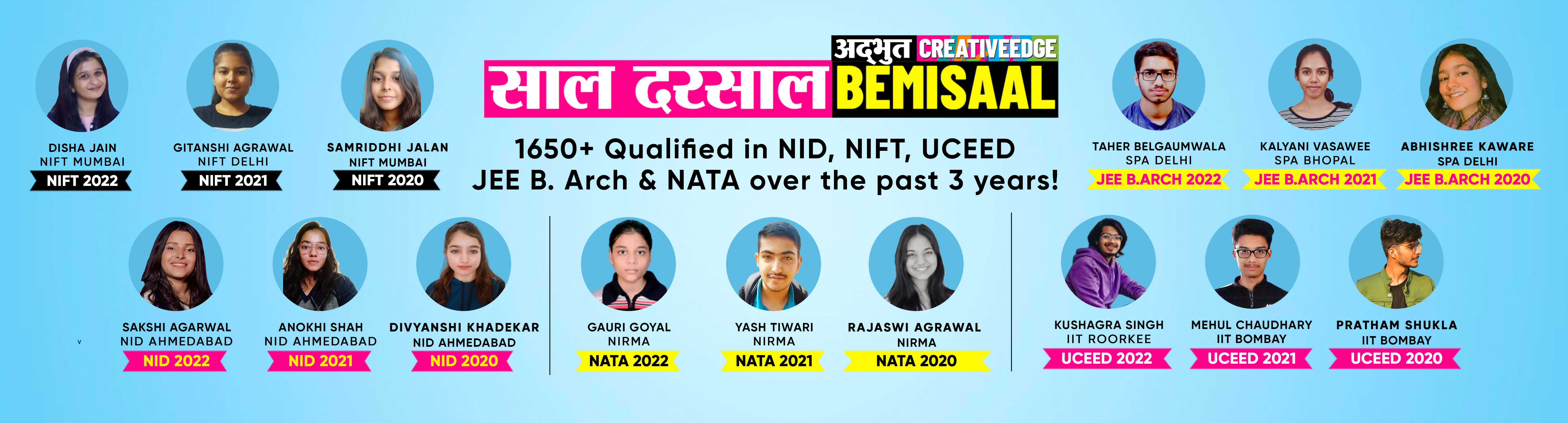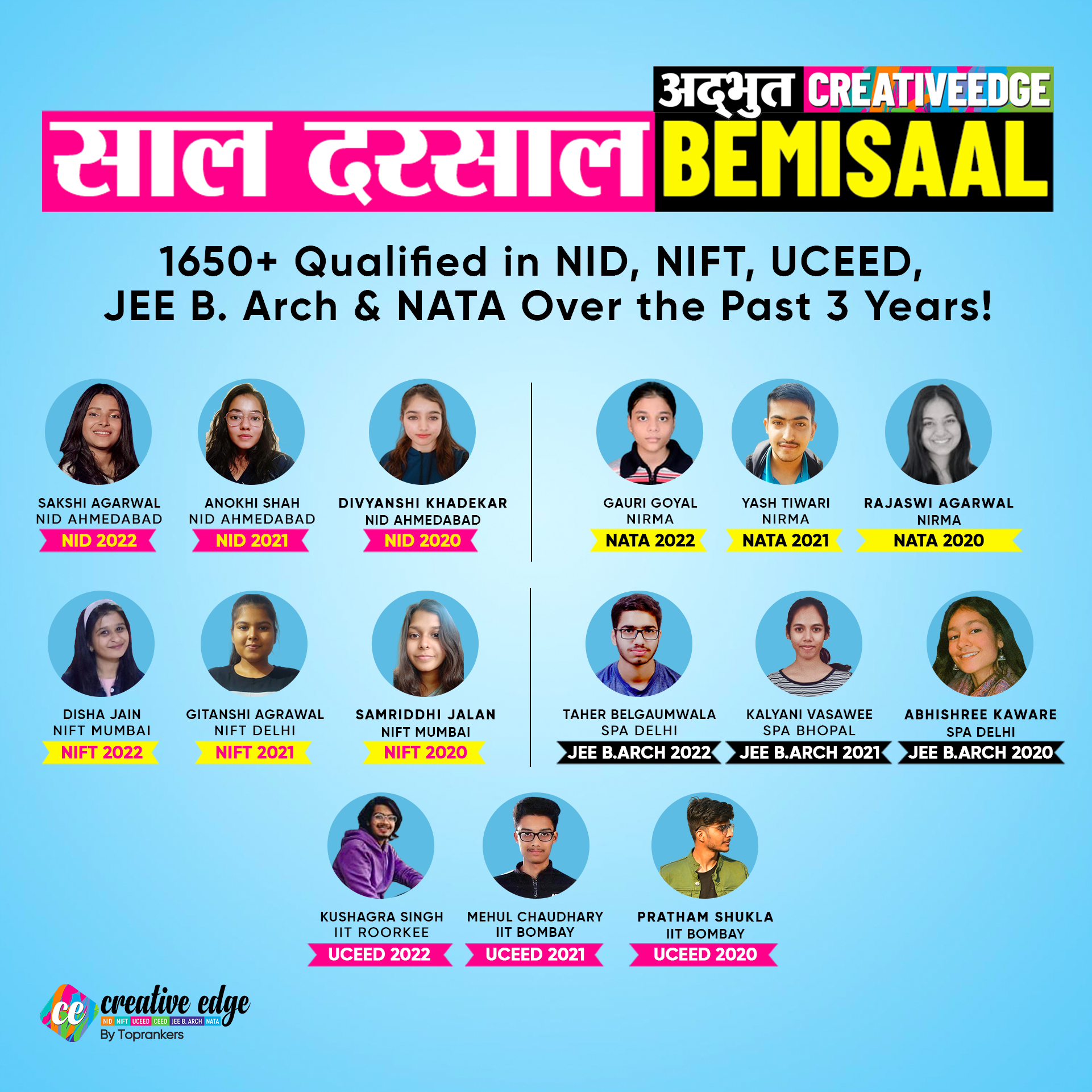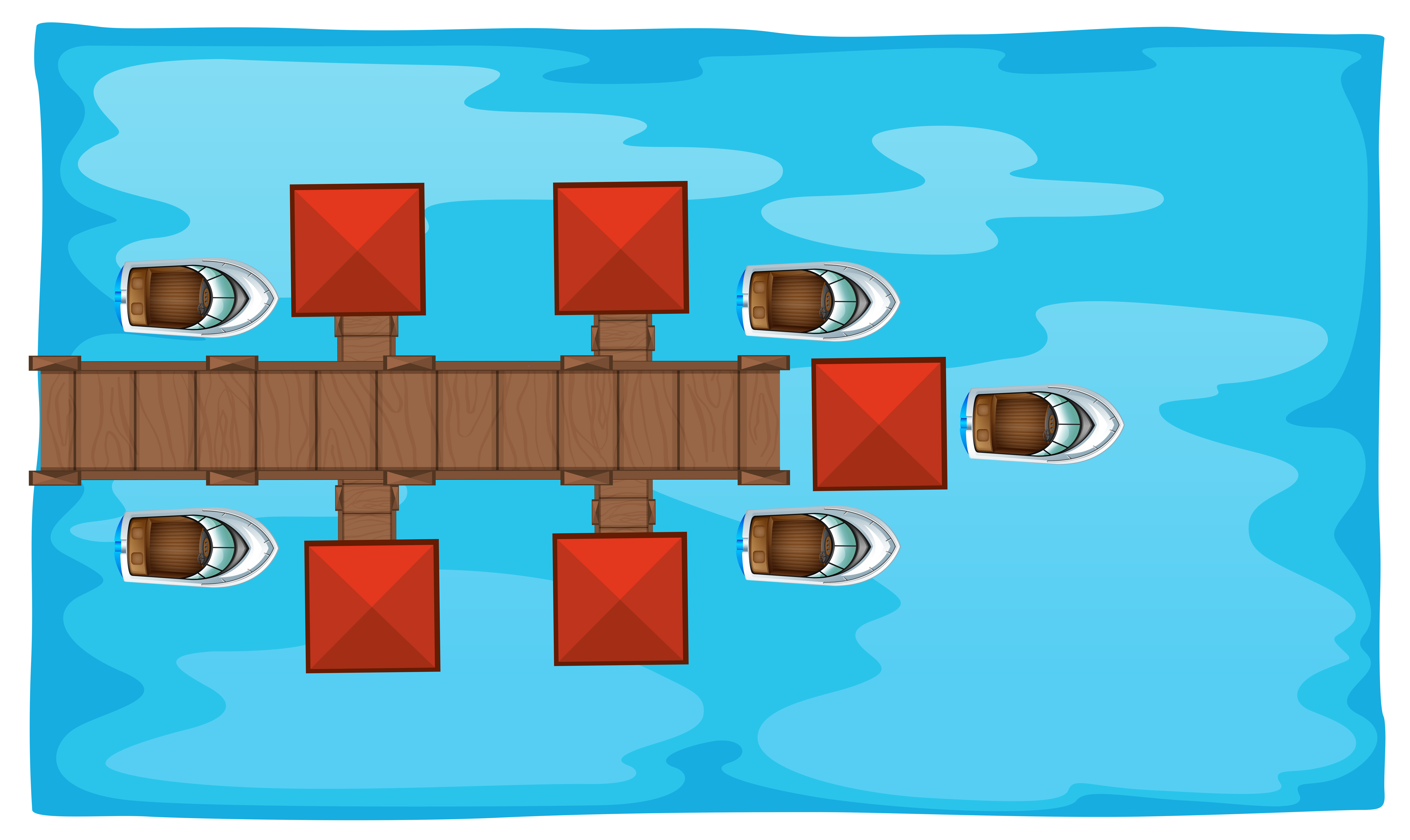• Home
• Aptitude Preparation...

# Aptitude Preparation - Important Formulae to Crack Design Entrance Exams 2024

Author : Reeshav Guha

Updated On : July 26, 2023

SHARE

Summary: The topic of the entrance examination syllabus naturally comes up when talking about design entrance exams like NATA and NIFT, UCEED, or any other entrance examination of a private college for B.Des or other design programmes. Numerous of you have inquired about how to prepare for the design aptitude test; therefore, we have made the decision to provide you with some brief tips to handle this section.

We can now share the good news with you once we've started responding to your questions about the examinations. We're going to go over some helpful hints and shortcuts in this blog that you may utilise to navigate the questions in your aptitude portion.

Aptitude sections usually have maths, qualitative and quantitative reasoning, which students require to solve to gain points.

However, due to the strict time limit, you might get panicked. In such a situation, you can do the whole calculation wrong, which will lead you to mark the wrong answer choice.

In return, you will have to have a negative marking. To eliminate this possibility, you just know the shortcuts, and here we are sharing them with you. Let's check the Important Formulae to Crack Design Entrance Exams 2024!

## Important Formulae to Crack Design Entrance Exams 2024: Speed, time, and distance

It is the first topic that is generalized and you will find Aptitude questions based on this concept in every design examination. This might come across as the most basic topic but it has its struggle. It often creates confusion about how you should calculate your time and distance. In km/hr or m/sec. Whatever the case. You can use both formulas at your convenience. Let us share some illustrations and basic formulas with you for the same.

So,

Speed = D/T

The distance can be donated with km or m.

Time can be denoted with h (hour)/ sec (minutes).

Hence two formulas arise to calculate the speed of an object:

1. km/h
2. m/sec

Therefore,

1km/hr = 1000m / 60x 60 sec

= 5/18 m/sec

Now let's look into the application of these formulas so that you don't get confused while attempting similar questions in the Examination.

Example: Convert 36km/h into m/sec.

Ans: 1 hour/km = 5/18 m/sec

Hence,

= 36x5/18 m/sec

= 10m/secLet's look into another example.

Eg: What is 18m/sec

Now,

1km/h = 5/18 m/sec

Then,

18/5km per hour = 1m/sec

= 18 x 18/5 km

= 324/5

= 64.8km/h

Now let's deal with a real-time question that you can encounter in the examination.

A man travels at ⅔ of his speed and reaches 30 min late to his destination. Find the time if he travels at the original speed.

Let the time be ⅔ x. Now,

x km/h = ⅔ x km/h

X x t = ⅔ x ( t + 30)

X x t = ⅔ x ( t + 30/60)

t = ⅔ t +( ½ x ⅔ )

t - ⅔ t = ⅓

t/3 = ⅓

T = 1 hour.

You can also solve this equation without converting 30 mins into seconds. Let us show you how.

X x t = ⅔ x ( t + 30)

t = ⅔ t + ⅔ x 30

t - ⅔ t = ⅔ x 30

t/3 = 20

t = 60 mins.

Read more: Best Books for Design Entrance Exams

## Important Formulae to Crack Design Entrance Exams 2024: Speed, time, and distance in the context of train

Now let's look into the speed, distance, and time formula but here we will discuss the problems based on trains. These questions are slightly different from the regular speed distance and time calculation because we have to consider the length of the train while calculating the sums. We will show you how to solve it with the help of the illustration below.Illustration: Train A, length of 600m, running at speed of 80 km/hr will take how long to traverse a man seated in the second train having a length of 400 meters, going at 64 km/h in the opposing path?

Ans:

Distance = six hundred meters

Total Speed = 64 + 80 = one hundred forty-four kmph (delivered due to the fact they're visiting in contrary directions)

In m/sec, speed = one hundred forty-four *(5/18) = forty m/sec

Distance = Speed * Time

six hundred = forty * Time

Hence, Time = 15 seconds

Notes: Important formulas.

1. i) Time when a train passes and objects at a certain speed =

length of a train

______________

Speed of a train.

1. ii) Thickness of the object =

length of a train + length of the platform

_______________________________

Speed of the train

Time is taken by a train moving in opposite directions:

L1 + L2

______

S1 + S2

{L stands for the length of the train while S stands for the speed of the train.}

Time is taken by a train moving in the same directions

L1 + L2

______

S1 - S2

## Boats and Stream

Boats and streams are another set of problems where your speed distance and time formula is applicable. However, the application here differs from train-based reasoning. How? Let's see.So basically Boats and streams have subparts when it comes to the classification of possible questions which you might face in the examination. Those are :

1. Stream
2. Standing Water
3. Upstream
4. Downstream

To solve questions for each type there are different formulas applicable. If you get them right then you will be able to solve these questions in no time.

Upstream: {u-v} km/h

Downstream: { u + v} km/h

Boat Speed in standing water: ½ upstream + downstream

stream speed: ½ {downstream - Upstream}

Boats Avg Speed: downstream x Upstream/boat speed in standing water## Important Formulae to Crack Design Entrance Exams 2024: Time and Work

Time and work-based reasoning are the other common questions that test your IQ as well as your patience in the examination. Although solving the question based on time and work is not a big deal in the regular time-space. During the examination, it could haunt you because of lengthy questions and limited time. However, you can Master this segment too if you know the shortcuts of solving these time and work reasoning and have applied them in your practice paper and mock test. Let us walk you through the formulas for solving time and Work-based reasonings:

{ Work Done} = Time Taken × Rate of Work

{Rate of Work} = 1 / Time Taken

{Total Time Taken} = 1 / Rate of Work

Assume a portion of work is accomplished in x digit of days, then the work accomplished in 1 day = { 1/x}

Aggregate Work accomplished = {No of Days × Efficiency}

Efficiency and Time are inversely proportional to each other

The simplest formula applicable when the question is asking about the aggregate work done by a certain number of people in a certain time, then the formula will be;

M1 x D1 x T1 M2 x D2 x T2

___________ = ___________

W1 W2

That was it for this blog. In the next blog, we will come up with another set of shortcuts and formulas to aid and enhance your Aptitude section preparation. Till then you can work on mastering these questions.

How do I participate in the Architecture & Design Online Coaching classes? Can I use my mobile to access classes?How can I make the payment for Architecture & Design Online Coaching?What are the technical requirements needed to attend the Architecture & Design Online Coaching?What are some of the topics of importance in the Design Aptitude Examination?How should we do our design Aptitude test preparation?Is it important to acquire information about mathematical formulas to solve reasoning questions?From where I can get the aptitude reasonings formulas pdf?Why is it important to learn Formulae for dealing with the Design Aptitude Test?